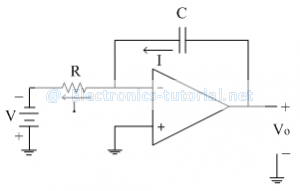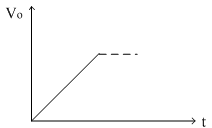# Triangular waveform generator

The square and triangular waveform generator has been discussed in previous section with the necessary waveform and equations.
To generate the triangular wave, a basic integrator circuit is used with step signal as an input is shown in figure below.Vo=-1/RC ∫(-V) dt
∴Vo=V/RC (t)
Thus output voltage increases linearly with time 't'; but after some time it goes into saturation as shown below.The current flowing through resistor 'R' is
I= V/R
As voltage 'V' and 'R' both are constant, current 'I' is also constant. This constant current 'I' is also flowing through capacitor C. When capacitor charges with current 'I'; it is called as constant current charging. When capacitor is charged up to constant current, voltage across capacitor is linearly increased with time i.e. ramp signal.
∴Vo=V/RC (t)
∴Vo=I/C (t)
If current 'I' is large, slope of ramp is large. If current 'I' is small, slope of ramp is small.